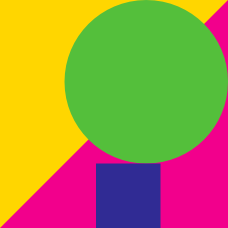Geometry

# Angles and Shapes Warmup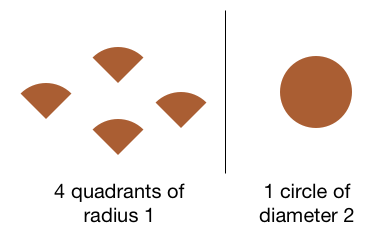Can we use each of the shapes on the left exactly once to make the shape on the right without any cutting?

(The radius of the wedges are each 1 unit, and the diameter of the circle is 2 units.)

###### Bonus: If your answer is no, can you prove that it cannot be done?

What is the maximum number of congruent equilateral triangles that all meet at a point without overlapping? (Touching at edges is still allowed.)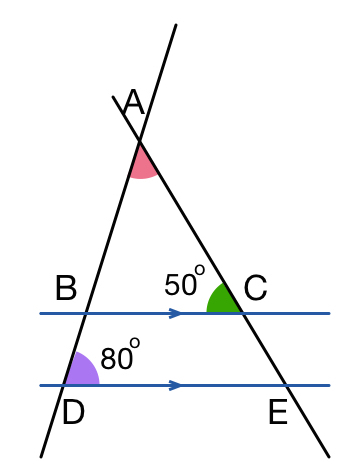$\overline{BC}$ and $\overline{DE}$ are parallel lines.
The green angle is $50 ^ \circ$ and the purple angle is $8 0 ^ \circ$.

What is the red angle ( $\angle DAE$ ) in degrees?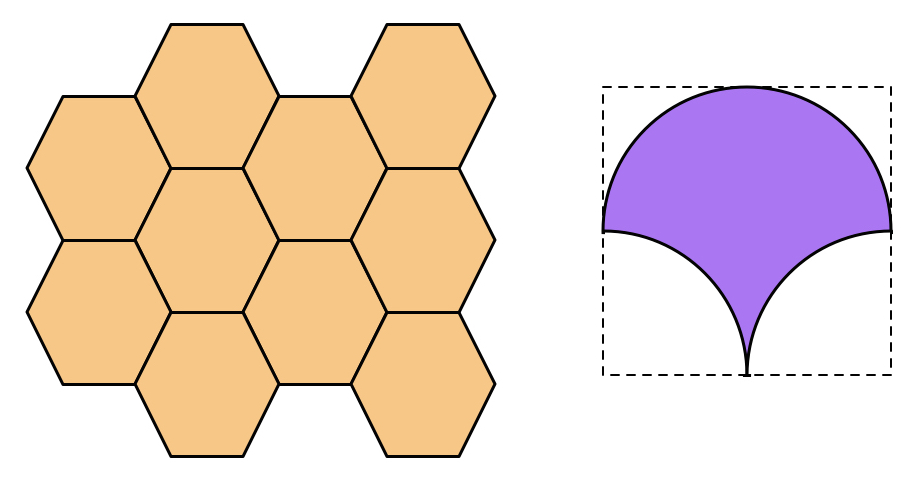The image on the left shows how hexagons could be used to cover the plane without leaving gaps or overlapping each other.

Can copies of the purple region on the right be arranged to cover the plane without leaving gaps or overlapping each other?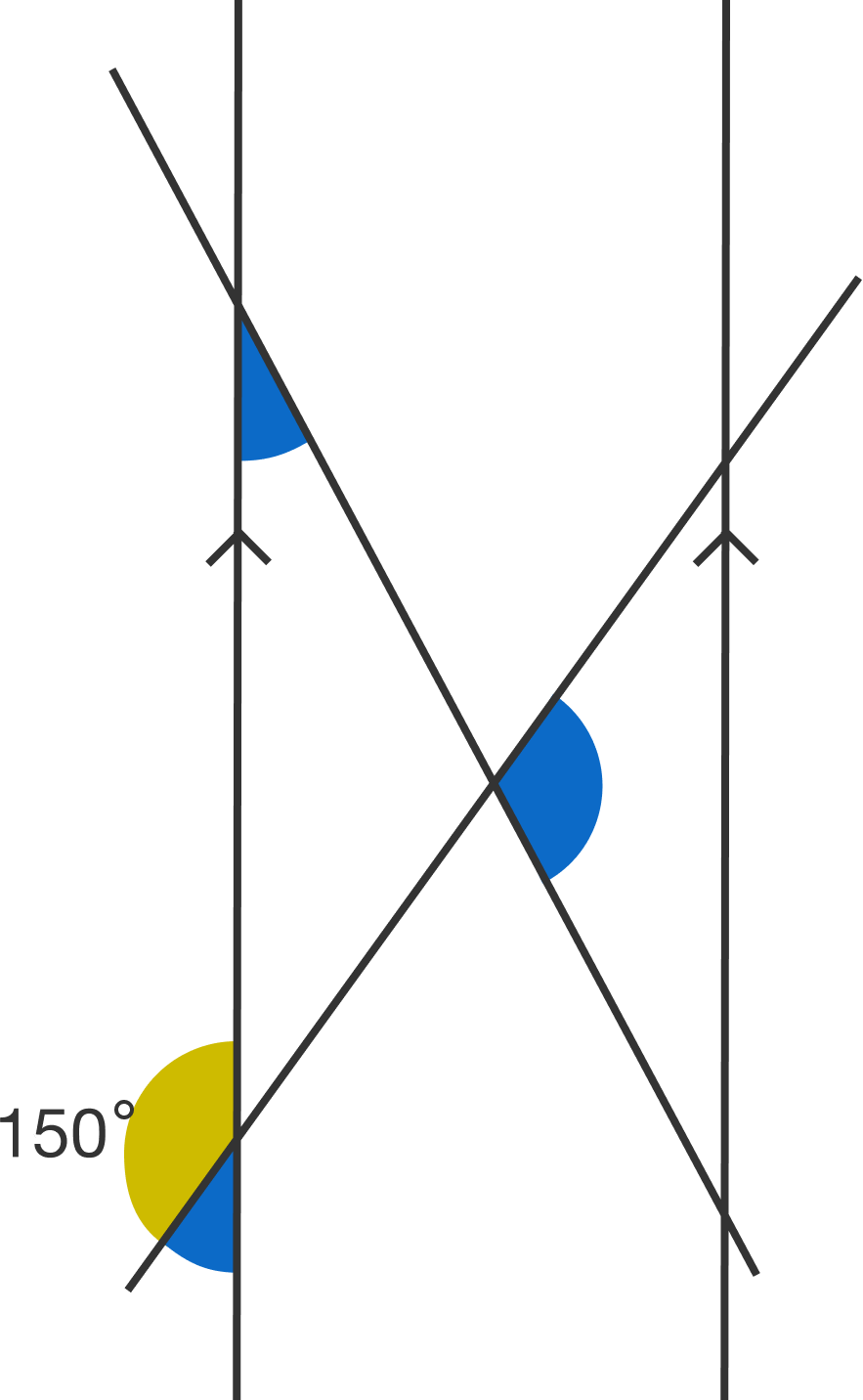The two vertical lines are perfectly parallel. What is the sum of the three blue angles?

×